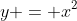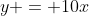## Monday, June 13, 2011

### Game Theory (Part 7) - Functions, Limits and Derivatives

This post is part of my course on game theory. For an index see here. The course follows the one by Ben Polak on the Open Yale website.

Over the last few posts we’ve been working our way through the concept of best response. We’ve now reached an impasse: to take our analysis to the next level we’ll need to know how to find the maximum value of a function. This requires some elementary calculus. Some readers might be fluent in this area of mathematics. If so, they can skip the next few parts (there will probably be three of them). For those who aren’t au fait with calculus, I’m going to quickly run through the basics and provide links to some other resources.

Listen, I’m not particularly comfortable with calculus myself, and I certainly wouldn’t offer myself up as an internet oracle on this topic. But the level of calculus required for the types of game theory problem covered in this course is pretty low. If I can understand it, anyone can.

(Oh and incidentally, if there are any calculus gurus reading this, they might be kind enough to let me know if I make a mistake.)

1. What is a function?
Since our goal is to learn how to find the maximum value of a function, we must first ask: what is a function? This needn’t detain us too long. A function is simply a mathematical way of depicting the relationship between two variables: an independent variable (usually denoted by an “x”) and a dependent variable (usually denoted by a “y” or “f(x)”).

As the value of the independent variable changes, so too does the value of the dependent variable. In other words, every value of x is associated with one value of y. The domain of a function is the set of all x where the function can be meaningfully applied. The range of a function is the set of y or f(x) values that result.

Here’s an example:According to this function, as the value of x increases (or decreases) the value of y increases in such a way that it is equal to the square of the x value. This function can be depicted graphically as follows.

Here’s another function:According to this function, every unit increase in x leads to ten unit increase in y. This can be depicted graphically as follows:

2. What is a Limit?
So much for functions, now we move on to limits. Here’s a classic example of a limit in action:

Achilles wants to cross the road. The road is 10m wide. Before he does so, a rather annoying tortoise waddles up beside him and tells him its impossible to cross the road. The tortoise reasons as follows: in order to cross a road which is 10m in width, Achilles will first have to cross half that distance (5m); and in order to cross the remaining distance of 5m, Achilles will first have to cross half that distance (2.5m); and of course in order to cross the remaining 2.5m after that, he’ll first have to cross 1.25m; and so on ad infinitum. Since there are infinite number of half distances to cross, and since it is impossible to cross an infinite space, Achilles will never be able to cross the road.

What we have here is a limit. There is an infinite series (5 + 2.5 + 1.25.....) which has a limiting value of 10. By repeatedly crossing half distances, Achilles gets closer and closer to the full 10m distance, but never quite reaches it.

Although a little weird, limits are mathematically tractable. Indeed, when calculating the derivative of a function -- something that must be done when finding its maximum value -- one is finding a limit of a certain sort.

3. What is a Derivative?
Finally, we have the concept of a derivative. Put simply, a derivative is the rate of change of a function. More properly, it’s the rate at which the dependent variable changes with respect to the rate at which the independent variable changes. It’s easiest to understand this with an example in mind. And an easy example to work with is the y = 10x one from above.

Imagine this function depicts a runner (like Achilles) running along a road. The independent variable here is time and it is represented on the x-axis; the dependent variable is distance travelled and it is represented on the y-axis. Now let’s ask the question: what is the rate of change of y with respect to x? The answer, obviously, is 10: there are ten unit changes in y with respect. This is the derivate of this particular function.

That was pretty easy. An even easier example would be where the function depicts a runner who is standing still 10 meters from the start line. In this case y = 10 at all times. And so the dependent variable does not change at all with respect to the independent variable. Consequently, the derivative of this function is zero. There is a generalisable lesson from this as well: the derivate of any constant is always equal to zero.

A far more interesting derivative is that which can be calculate the accelerating force of gravity. This is discussed at length in the second episode of the 1980s TV series The Mechanical Universe . You can find it here.

Okay, that’s it for now. In the next part, we’ll go through the basic method for calculating a derivative in more detail.

Resources
In terms of books, I would recommend Calculus Made Easyby Silvanus Thompson. This is a classic text that was updated a few years back by Martin Gardner. It focuses heavily on facilitating comprehension of calculus, i.e explaining the rationale behind the various calculations. Most of the examples used in this post were taken from this book. I’d also recommend the Schaum’s Outlinesbooks dealing with calculus. They focus more on practical applications and problem-solving.

In terms of videos, Salman Khan has quite a number of tutorials on calculus over at the Khan Academy website. I also found the youtube series by the Calculus Tutor to be helpful.